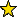# ExteriorIdeals -- a package for working with ideals over exterior algebra

## Description

ExteriorIdeals is a package for creating and manipulating ideals over exterior algebra

Other acknowledgements:

The method isLexIdeal was taken from Chris Francisco's package: LexIdeals, which is available at LexIdeals

## CertificationVersion 1.0 of this package was accepted for publication in volume 8 of The Journal of Software for Algebra and Geometry on 24 June 2018, in the article ExteriorIdeals: a package for computing monomial ideals in an exterior algebra. That version can be obtained from the journal or from the Macaulay2 source code repository.

## Version

This documentation describes version 1.1 of ExteriorIdeals.

## Source code

The source code from which this documentation is derived is in the file ExteriorIdeals.m2.

## Exports

• Functions and commands
• allHilbertSequences -- compute all Hilbert sequences of quotients in an exterior algebra
• hilbertSequence -- compute the Hilbert sequence of a given ideal in an exterior algebra
• initialDegree -- compute the initial degree of a graded ideal
• initialIdeal -- compute the initial ideal of a given ideal
• isHilbertSequence -- whether the given sequence is a Hilbert sequence
• isLexIdeal -- whether an ideal is lex
• isStableIdeal -- whether a monomial ideal in an exterior algebra is stable
• isStronglyStableIdeal -- whether a monomial ideal in an exterior algebra is strongly stable
• lexIdeal -- compute the lex ideal with a given Hilbert function in an exterior algebra
• macaulayExpansion -- compute the Macaulay expansion of a positive integer
• minimalBettiNumbers -- compute the minimal Betti numbers of a given graded ideal
• solveMacaulayExpansion -- compute the sum of a Macaulay expansion
• stableIdeal -- compute the smallest stable ideal in an exterior algebra containing a given monomial ideal
• stronglyStableIdeal -- compute the smallest strongly stable ideal in an exterior algebra containing a given monomial ideal
• Methods
• "allHilbertSequences(Ring)" -- see allHilbertSequences -- compute all Hilbert sequences of quotients in an exterior algebra
• "hilbertSequence(Ideal)" -- see hilbertSequence -- compute the Hilbert sequence of a given ideal in an exterior algebra
• "initialDegree(Ideal)" -- see initialDegree -- compute the initial degree of a graded ideal
• "initialIdeal(Ideal)" -- see initialIdeal -- compute the initial ideal of a given ideal
• "isHilbertSequence(List,Ring)" -- see isHilbertSequence -- whether the given sequence is a Hilbert sequence
• "isLexIdeal(Ideal)" -- see isLexIdeal -- whether an ideal is lex
• "isStableIdeal(Ideal)" -- see isStableIdeal -- whether a monomial ideal in an exterior algebra is stable
• "isStronglyStableIdeal(Ideal)" -- see isStronglyStableIdeal -- whether a monomial ideal in an exterior algebra is strongly stable
• "lexIdeal(Ideal)" -- see lexIdeal -- compute the lex ideal with a given Hilbert function in an exterior algebra
• "lexIdeal(List,Ring)" -- see lexIdeal -- compute the lex ideal with a given Hilbert function in an exterior algebra
• "macaulayExpansion(ZZ,ZZ)" -- see macaulayExpansion -- compute the Macaulay expansion of a positive integer
• "minimalBettiNumbers(Ideal)" -- see minimalBettiNumbers -- compute the minimal Betti numbers of a given graded ideal
• "solveMacaulayExpansion(List)" -- see solveMacaulayExpansion -- compute the sum of a Macaulay expansion
• "stableIdeal(Ideal)" -- see stableIdeal -- compute the smallest stable ideal in an exterior algebra containing a given monomial ideal
• "stronglyStableIdeal(Ideal)" -- see stronglyStableIdeal -- compute the smallest strongly stable ideal in an exterior algebra containing a given monomial ideal
• Symbols
• Shift -- optional argument for macaulayExpansion

## For the programmer

The object ExteriorIdeals is .